# 我又闲下来了，再做论坛网络状态测试~~~参与者都有分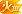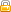[复制链接]
 正在 Ping pceva.3322.org [180.166.1.70] 具有 32 字节的数据: 来自 180.166.1.70 的回复: 字节=32 时间=24ms TTL=243 来自 180.166.1.70 的回复: 字节=32 时间=25ms TTL=243 来自 180.166.1.70 的回复: 字节=32 时间=36ms TTL=243 来自 180.166.1.70 的回复: 字节=32 时间=40ms TTL=243 来自 180.166.1.70 的回复: 字节=32 时间=24ms TTL=243 来自 180.166.1.70 的回复: 字节=32 时间=24ms TTL=243 来自 180.166.1.70 的回复: 字节=32 时间=25ms TTL=243 来自 180.166.1.70 的回复: 字节=32 时间=25ms TTL=243 来自 180.166.1.70 的回复: 字节=32 时间=25ms TTL=243 来自 180.166.1.70 的回复: 字节=32 时间=25ms TTL=243 来自 180.166.1.70 的回复: 字节=32 时间=24ms TTL=243 来自 180.166.1.70 的回复: 字节=32 时间=24ms TTL=243 来自 180.166.1.70 的回复: 字节=32 时间=25ms TTL=243 来自 180.166.1.70 的回复: 字节=32 时间=25ms TTL=243 来自 180.166.1.70 的回复: 字节=32 时间=25ms TTL=243 来自 180.166.1.70 的回复: 字节=32 时间=25ms TTL=243 来自 180.166.1.70 的回复: 字节=32 时间=25ms TTL=243 来自 180.166.1.70 的回复: 字节=32 时间=25ms TTL=243 来自 180.166.1.70 的回复: 字节=32 时间=25ms TTL=243 来自 180.166.1.70 的回复: 字节=32 时间=26ms TTL=243 180.166.1.70 的 Ping 统计信息:     数据包: 已发送 = 20，已接收 = 20，丢失 = 0 (0% 丢失)， 往返行程的估计时间(以毫秒为单位):     最短 = 24ms，最长 = 40ms，平均 = 26ms

### 评分

 我又来了，湖北移动2g网络，纯骗分←_← \$ ping -n 20 bbs.pceva.com.cn connect: Invalid argument \$ ping -n 20 bbs.pceva.com.cn connect: Invalid argument \$ ping -c 20 bbs.pceva.com.cn PING pceva.3322.org (180.166.1.70) 56(84) bytes of data. 64 bytes from 180.166.1.70: icmp_seq=1 ttl=238 time=493 ms 64 bytes from 180.166.1.70: icmp_seq=2 ttl=238 time=280 ms 64 bytes from 180.166.1.70: icmp_seq=3 ttl=238 time=299 ms 64 bytes from 180.166.1.70: icmp_seq=4 ttl=238 time=320 ms 64 bytes from 180.166.1.70: icmp_seq=5 ttl=238 time=255 ms 64 bytes from 180.166.1.70: icmp_seq=6 ttl=238 time=277 ms 64 bytes from 180.166.1.70: icmp_seq=7 ttl=238 time=296 ms 64 bytes from 180.166.1.70: icmp_seq=8 ttl=238 time=280 ms 64 bytes from 180.166.1.70: icmp_seq=9 ttl=238 time=259 ms 64 bytes from 180.166.1.70: icmp_seq=10 ttl=238 time=296 ms 64 bytes from 180.166.1.70: icmp_seq=11 ttl=238 time=277 ms 64 bytes from 180.166.1.70: icmp_seq=12 ttl=238 time=274 ms 64 bytes from 180.166.1.70: icmp_seq=13 ttl=238 time=293 ms 64 bytes from 180.166.1.70: icmp_seq=14 ttl=238 time=297 ms 64 bytes from 180.166.1.70: icmp_seq=15 ttl=238 time=297 ms 64 bytes from 180.166.1.70: icmp_seq=16 ttl=238 time=297 ms 64 bytes from 180.166.1.70: icmp_seq=17 ttl=238 time=294 ms 64 bytes from 180.166.1.70: icmp_seq=18 ttl=238 time=316 ms 64 bytes from 180.166.1.70: icmp_seq=19 ttl=238 time=293 ms 64 bytes from 180.166.1.70: icmp_seq=20 ttl=238 time=254 ms --- pceva.3322.org ping statistics --- 20 packets transmitted, 20 received, 0% packet loss, time 19199ms rtt min/avg/max/mdev = 254.013/297.780/493.981/48.291 ms \$

### 评分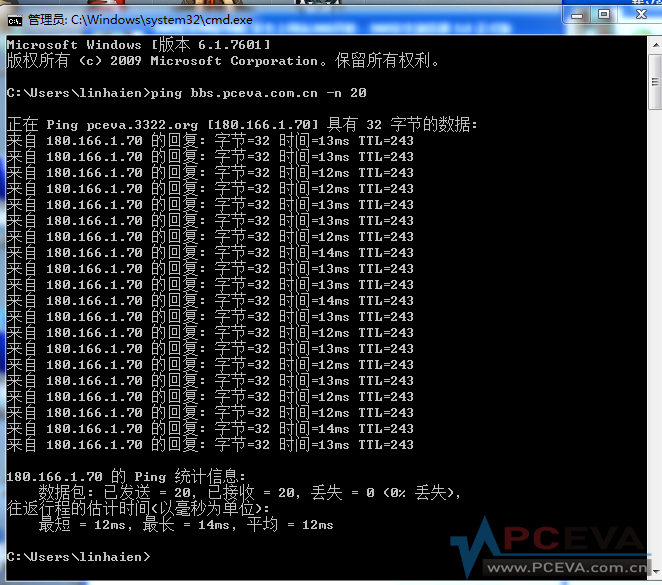温州电信。

### 评分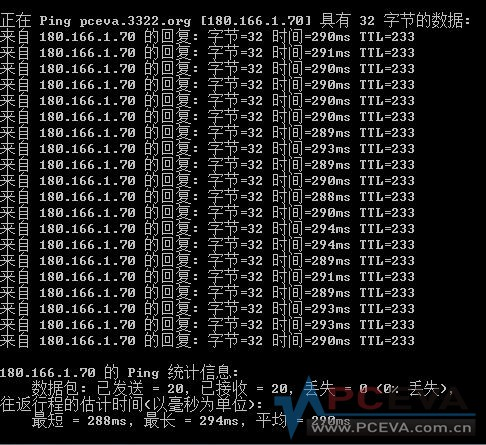### 评分

 rkingj 发表于 2012-8-17 12:17看来菠萝采纳了我的建议，把默认解析指向了上海服。正在 Ping pceva.3322.org [180.166.1.70] 具有 32 字节的数据: 来自 180.166.1.70 的回复: 字节=32 时间=77ms TTL=243 来自 180.166.1.70 的回复: 字节=32 时间=75ms TTL=243 来自 180.166.1.70 的回复: 字节=32 时间=76ms TTL=243 来自 180.166.1.70 的回复: 字节=32 时间=76ms TTL=243 来自 180.166.1.70 的回复: 字节=32 时间=76ms TTL=243 来自 180.166.1.70 的回复: 字节=32 时间=76ms TTL=243 来自 180.166.1.70 的回复: 字节=32 时间=75ms TTL=243 来自 180.166.1.70 的回复: 字节=32 时间=76ms TTL=243 来自 180.166.1.70 的回复: 字节=32 时间=77ms TTL=243 来自 180.166.1.70 的回复: 字节=32 时间=76ms TTL=243 来自 180.166.1.70 的回复: 字节=32 时间=76ms TTL=243 来自 180.166.1.70 的回复: 字节=32 时间=76ms TTL=243 来自 180.166.1.70 的回复: 字节=32 时间=77ms TTL=243 来自 180.166.1.70 的回复: 字节=32 时间=76ms TTL=243 来自 180.166.1.70 的回复: 字节=32 时间=77ms TTL=243 来自 180.166.1.70 的回复: 字节=32 时间=76ms TTL=243 来自 180.166.1.70 的回复: 字节=32 时间=75ms TTL=243 来自 180.166.1.70 的回复: 字节=32 时间=77ms TTL=243 来自 180.166.1.70 的回复: 字节=32 时间=76ms TTL=243 来自 180.166.1.70 的回复: 字节=32 时间=76ms TTL=243 180.166.1.70 的 Ping 统计信息:     数据包: 已发送 = 20，已接收 = 20，丢失 = 0 (0% 丢失)， 往返行程的估计时间(以毫秒为单位):     最短 = 75ms，最长 = 77ms，平均 = 76ms

### 评分

 一下就没了，怎么截图，复制呢？
 zxy356 发表于 2012-8-17 12:40一下就没了，怎么截图，复制呢？ 点开始按钮，在命令行框里输入cmd，回车，在打开的小黑窗中输入ping bbs.pceva.com.cn -n 20 如何复制？ 点小黑窗左上角，菜单中选 编辑，再选 标记，按住鼠标拖选要复制区域，完成后按回车，就复制到了剪贴板中，回到论坛粘贴即可。

### 评分

 上海电信  公司10M光纤 汇报成绩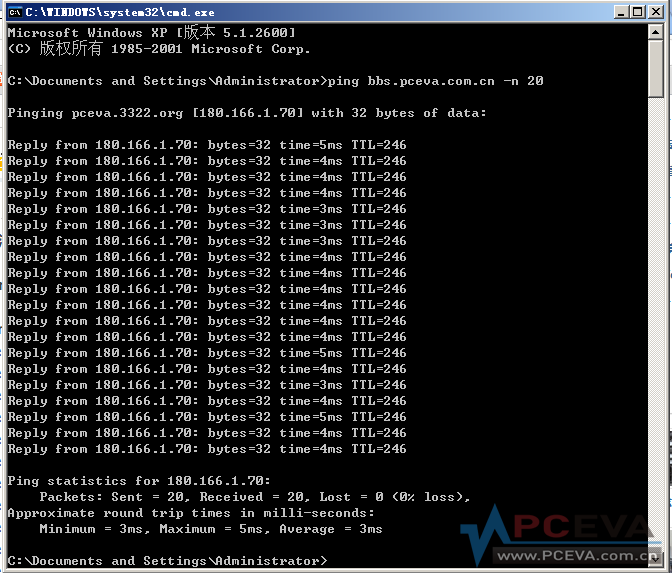### 评分

 本帖最后由 诚实的旦旦 于 2012-8-17 13:41 编辑 看出我的网络质量还可以，5M小管三台手机两台电脑同时在线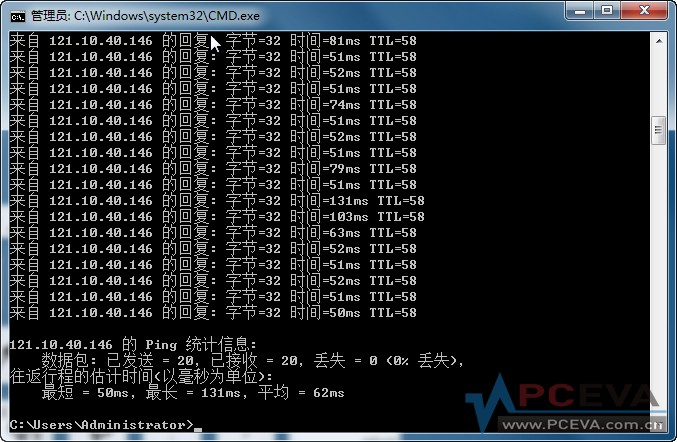### 评分

 本帖最后由 wsy2220 于 2012-8-17 13:44 编辑 再来一个，电信adsl \$  ping -c 20 bbs.pceva.com.cn PING pceva.3322.org (180.166.1.70) 56(84) bytes of data. 64 bytes from 180.166.1.70: icmp_seq=1 ttl=244 time=47.7 ms 64 bytes from 180.166.1.70: icmp_seq=2 ttl=244 time=53.2 ms 64 bytes from 180.166.1.70: icmp_seq=3 ttl=244 time=61.2 ms 64 bytes from 180.166.1.70: icmp_seq=4 ttl=244 time=48.0 ms 64 bytes from 180.166.1.70: icmp_seq=5 ttl=244 time=59.0 ms 64 bytes from 180.166.1.70: icmp_seq=6 ttl=244 time=55.7 ms 64 bytes from 180.166.1.70: icmp_seq=7 ttl=244 time=47.1 ms 64 bytes from 180.166.1.70: icmp_seq=8 ttl=244 time=53.7 ms 64 bytes from 180.166.1.70: icmp_seq=9 ttl=244 time=62.6 ms 64 bytes from 180.166.1.70: icmp_seq=10 ttl=244 time=62.2 ms 64 bytes from 180.166.1.70: icmp_seq=11 ttl=244 time=58.6 ms 64 bytes from 180.166.1.70: icmp_seq=12 ttl=244 time=55.5 ms 64 bytes from 180.166.1.70: icmp_seq=13 ttl=244 time=54.0 ms 64 bytes from 180.166.1.70: icmp_seq=14 ttl=244 time=53.4 ms 64 bytes from 180.166.1.70: icmp_seq=15 ttl=244 time=59.6 ms 64 bytes from 180.166.1.70: icmp_seq=16 ttl=244 time=58.6 ms 64 bytes from 180.166.1.70: icmp_seq=17 ttl=244 time=58.8 ms 64 bytes from 180.166.1.70: icmp_seq=18 ttl=244 time=59.6 ms 64 bytes from 180.166.1.70: icmp_seq=19 ttl=244 time=57.5 ms 64 bytes from 180.166.1.70: icmp_seq=20 ttl=244 time=53.3 ms --- pceva.3322.org ping statistics --- 20 packets transmitted, 20 received, 0% packet loss, time 19029ms rtt min/avg/max/mdev = 47.115/55.991/62.620/4.533 ms \$

### 评分

 杭州下沙电信ADSL..4M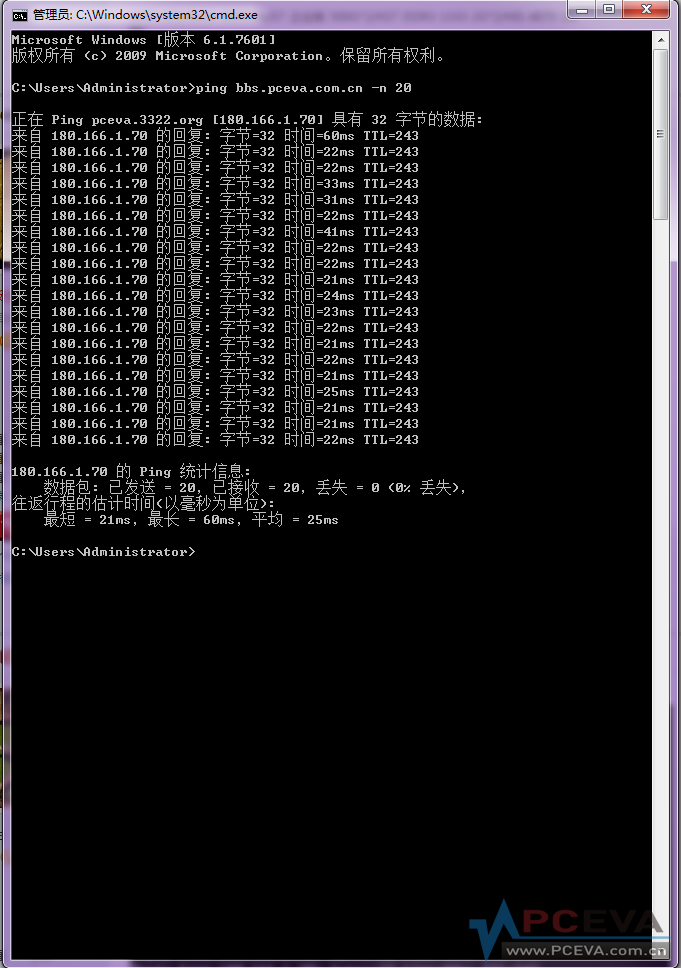### 评分

 北京联不通小区LAN 正在 Ping pceva.3322.org [180.166.1.70] 具有 32 字节的数据: 来自 180.166.1.70 的回复: 字节=32 时间=29ms TTL=242 来自 180.166.1.70 的回复: 字节=32 时间=30ms TTL=242 来自 180.166.1.70 的回复: 字节=32 时间=29ms TTL=242 来自 180.166.1.70 的回复: 字节=32 时间=29ms TTL=242 来自 180.166.1.70 的回复: 字节=32 时间=29ms TTL=242 来自 180.166.1.70 的回复: 字节=32 时间=29ms TTL=242 来自 180.166.1.70 的回复: 字节=32 时间=29ms TTL=242 来自 180.166.1.70 的回复: 字节=32 时间=29ms TTL=242 来自 180.166.1.70 的回复: 字节=32 时间=29ms TTL=242 来自 180.166.1.70 的回复: 字节=32 时间=29ms TTL=242 来自 180.166.1.70 的回复: 字节=32 时间=30ms TTL=242 来自 180.166.1.70 的回复: 字节=32 时间=30ms TTL=242 来自 180.166.1.70 的回复: 字节=32 时间=29ms TTL=242 来自 180.166.1.70 的回复: 字节=32 时间=29ms TTL=242 来自 180.166.1.70 的回复: 字节=32 时间=29ms TTL=242 来自 180.166.1.70 的回复: 字节=32 时间=29ms TTL=242 来自 180.166.1.70 的回复: 字节=32 时间=30ms TTL=242 来自 180.166.1.70 的回复: 字节=32 时间=29ms TTL=242 来自 180.166.1.70 的回复: 字节=32 时间=30ms TTL=242 来自 180.166.1.70 的回复: 字节=32 时间=29ms TTL=242 180.166.1.70 的 Ping 统计信息:     数据包: 已发送 = 20，已接收 = 20，丢失 = 0 (0% 丢失)， 往返行程的估计时间(以毫秒为单位):     最短 = 29ms，最长 = 30ms，平均 = 29ms 今天的真不错。

### 评分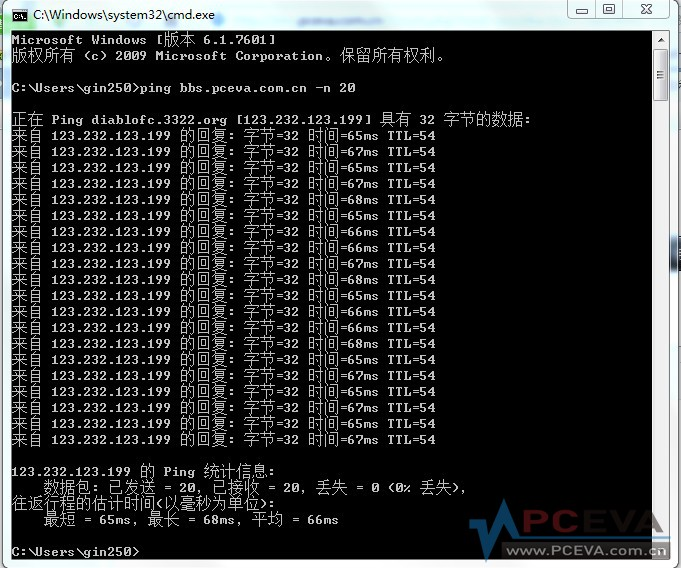辽宁网通的

### 评分

 江苏电信 ADSL 正在 Ping pceva.3322.org [180.166.1.70] 具有 32 字节的数据 来自 180.166.1.70 的回复: 字节=32 时间=17ms TTL=244 来自 180.166.1.70 的回复: 字节=32 时间=17ms TTL=244 来自 180.166.1.70 的回复: 字节=32 时间=17ms TTL=244 来自 180.166.1.70 的回复: 字节=32 时间=18ms TTL=244 来自 180.166.1.70 的回复: 字节=32 时间=18ms TTL=244 来自 180.166.1.70 的回复: 字节=32 时间=17ms TTL=244 来自 180.166.1.70 的回复: 字节=32 时间=18ms TTL=244 来自 180.166.1.70 的回复: 字节=32 时间=17ms TTL=244 来自 180.166.1.70 的回复: 字节=32 时间=17ms TTL=244 来自 180.166.1.70 的回复: 字节=32 时间=17ms TTL=244 来自 180.166.1.70 的回复: 字节=32 时间=18ms TTL=244 来自 180.166.1.70 的回复: 字节=32 时间=18ms TTL=244 来自 180.166.1.70 的回复: 字节=32 时间=17ms TTL=244 来自 180.166.1.70 的回复: 字节=32 时间=17ms TTL=244 来自 180.166.1.70 的回复: 字节=32 时间=18ms TTL=244 来自 180.166.1.70 的回复: 字节=32 时间=18ms TTL=244 来自 180.166.1.70 的回复: 字节=32 时间=17ms TTL=244 来自 180.166.1.70 的回复: 字节=32 时间=18ms TTL=244 来自 180.166.1.70 的回复: 字节=32 时间=17ms TTL=244 来自 180.166.1.70 的回复: 字节=32 时间=17ms TTL=244 180.166.1.70 的 Ping 统计信息:     数据包: 已发送 = 20，已接收 = 20，丢失 = 0 (0% 丢失)， 往返行程的估计时间(以毫秒为单位):     最短 = 17ms，最长 = 18ms，平均 = 17ms

### 评分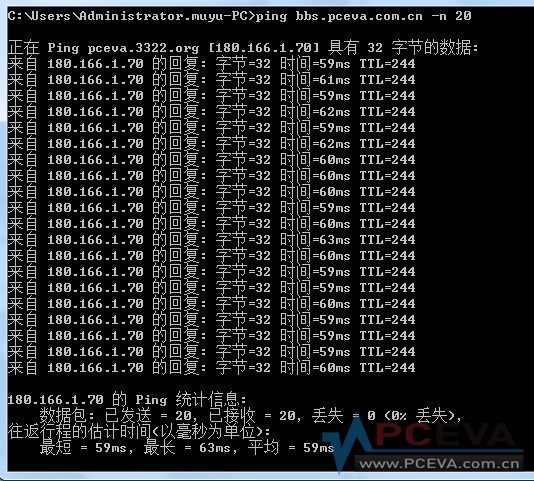四川电信 公司渣网络

### 评分